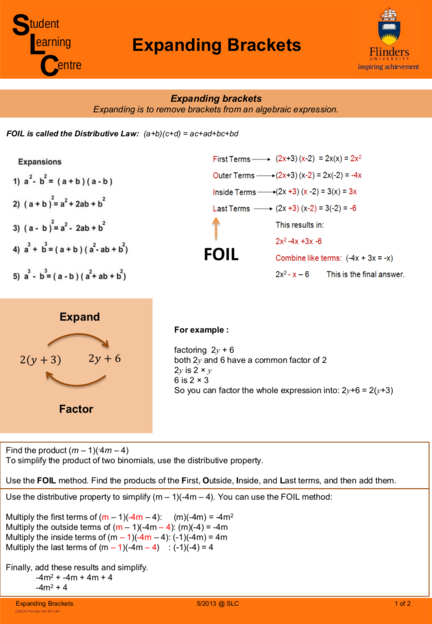# Expanding and Removing BracketsContributed by:This pdf includes the following topics:-
Expanding brackets
FOIL
Distributive Law
Distributive properties
Examples
1. Student
Learning Expanding Brackets
Centre
Expanding brackets
Expanding is to remove brackets from an algebraic expression.
FOIL is called the Distributive Law: (a+b)(c+d) = ac+ad+bc+bd
FOIL
Expand
For example :
factoring 2y + 6
2 𝑦𝑦 + 3 2𝑦𝑦 + 6 both 2y and 6 have a common factor of 2
2y is 2 × y
6 is 2 × 3
So you can factor the whole expression into: 2y+6 = 2(y+3)
Factor
Find the product (m – 1)(-4m – 4)
To simplify the product of two binomials, use the distributive property.
Use the FOIL method. Find the products of the First, Outside, Inside, and Last terms, and then add them.
Use the distributive property to simplify (m – 1)(-4m – 4). You can use the FOIL method:
Multiply the first terms of (m – 1)(-4m – 4): (m)(-4m) = -4m2
Multiply the outside terms of (m – 1)(-4m – 4): (m)(-4) = -4m
Multiply the inside terms of (m – 1)(-4m – 4): (-1)(-4m) = 4m
Multiply the last terms of (m – 1)(-4m – 4) : (-1)(-4) = 4
Finally, add these results and simplify.
-4m2 + -4m + 4m + 4
-4m2 + 4
Expanding Brackets 5/2013 @ SLC 1 of 2
2. DISTRIBUTIVE PROPERTIES
The Distributive Properties rules tell us that if you have a term being multiplied by two or more terms being
either added or subtracted within brackets (parenthesis), the term outside the brackets must be multiplied by
EVERY term within the brackets.
STUDENT LEARNING CENTRE
REGISTRY BUILDING ANNEXE
TEL: 61-8-8201 2518
E-MAIL: [email protected]
INTERNET: http://www.flinders.edu.au/SLC
POSTAL: PO BOX 2100, ADELAIDE, SA 5001
Expanding Brackets 5/2013 @ SLC 2 of 2